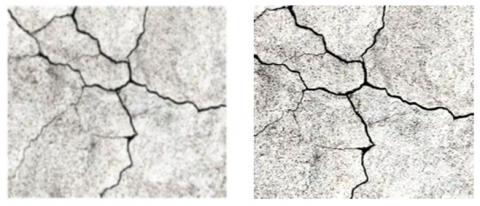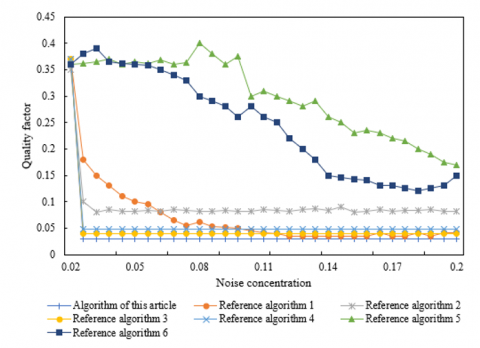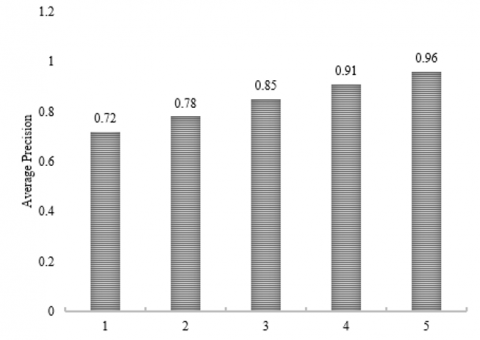# Research on the Real-Time Detection Method for Image Processing – Based Civil Structure Crack

Research on the Real-Time Detection Method for Image Processing – Based Civil Structure Crack
College of Engineering, Dali University, Dali 671003, China

Department of Civil Engineering, Army Logistics University of PLA, Chongqing 401331, China

Corresponding Author Email:
lwmofficial@163.com
Page:
2223-2228
|
DOI:
https://doi.org/10.18280/ts.390638
2 September 2022
|
Accepted:
5 December 2022
|
Published:
31 December 2022
| Citation

OPEN ACCESS

Abstract:

Early discovery of civil structure crack facilitates timely treatment, such as repair, reinforcement and grouting. The image processing – based rapid and accurate detection method for civil structure crack brings important research value. In view of complicated construction site of civil project and complex and diversified civil structure, existing method cannot realize crack detection and geometric measurement accurately. Therefore, this article researches the real-time image processing-based detection method for civil structure crack. Bilateral filtering algorithm was used to finish smoothing processing. Crack edge of civil structure was extracted with interpolation calculation – based subpixel edge detection algorithm, to obtain more accurate crack edge position. Civil structure crack was detected based on end-to-end object detection network YOLO. The effectiveness of the model built was verified according to experimental results.

Keywords:

image processing, civil structure crack, crack detection

1. Introduction

Hazard of crack is enormous [1-5] for civil projects. The reasons for cracks in civil structure mainly include load, temperature change, poor quality of construction materials and poor construction process quality. The problem breaks the original mechanical structure of civil project, affects durability of civil structure directly and further causes project safety quality problem. Therefore, the earlier the crack of civil structure is discovered, the better it will be, so that we could make repair, reinforcement and grouting timely [6-11]. With rapid development of image processing technology over the past several years, image processing-based crack detection for civil structure became a focus among scholars both at home and broad [12-14]. The research on rapid and accurate civil structure crack detection method has important research value.

A kind of high-calculation efficiency deep learning (DL) model for crack/non-crack real-time classification was discussed in Geetha and Sim , where scalable AI (XAI) was used to study “black box” nature of DL model raised. Integrated with image binarization and Fourier-based 1D DL model, the framework is used to detect and classify concrete crack/non-crack characteristics quickly. A weak supervision network to segment and detect asphalt concrete bridge deck crack was developed in Zhu and Song . First, data was distinguished through encoder and unmarked data characteristics were highlighted, so as to make original data generate weakly supervised convergence starting point. Second, characteristics were classified through K-means cluster (KMC). Then, semantic segmentation was implemented against crack in bridge deck defect image under weak supervision. According to experiment results, the method proposed could yield remarkable and better segmentation effect in all six types of defects, in comparison to other methods reported in references. A kind of DL object detection framework was proposed in Zhou et al.  by combining texture characteristics and concrete crack data. Texture characteristics and pre-processed concrete data were combined to increase quantity of characteristic channels, so as to lower demand for model training model and improve training speed. With this framework, concrete crack detection can be realized even if there are a limited number of samples available. A kind of analysis method was proposed in Xu et al.  to check crack development of glass fiber reinforced polymer (GFRP) - sea sand concrete composite material. The method is exported by analyzing strain dissipation and shear lag behavior and crack can be recognized quantitatively by the proposed Fourier transform analysis model. As shown by result, forecast error is less than 10%.

In view of complicated construction site of civil project and complex and diversified civil structure, existing method cannot realize crack detection and geometric measurement accurately. The main reason lies in the failure to pre-process civil structure crack through image processing technology and to extract crack intersection point steadily. For complicated and diversified civil structure, overfitting or underfitting problem easily occurs once the existing method is used, resulting in the failure to extract effective crack characteristic information. Therefore, this article researches the image processing-based real-time detection method for civil structure. The civil structure crack image was smoothed with bilateral filtering algorithm in Chapter 2 of this article. In Chapter 3, civil structure crack edge was extracted with interpolation calculation – based subpixel edge detection algorithm, so as to obtain more accurate crack edge position. After finishing pre-processing and edge extraction of civil structure crack image, civil structure crack was detected based on end-to-end object detection network YOLO in Chapter 4. The effectiveness of the model built was verified according to experimental results.

2. Image Pre-Processing of Civil Structure Crack

In reality, the acquired civil structure crack image contains many noisy points owing to multiple influence factors of light, temperature and acquisition equipment and these noisy points are unfavorable to classification of subsequent images and detection of civil structure crack. Therefore, civil structure crack image should be denoised prior to detection. In this article, bilateral filtering algorithm was used to smooth civil structure crack image.

Bilateral filtering means processing image through two gaussian filters. Suppose the gray information of crack image TX of civil structure in coordinate e=(i, j) is expressed as TXe, image obtained after filtration as TXS, gray value of image after filtration as TXYE, set of pixels in the neighborhood of filtered area as R, normalizing factor as QE and neighborhood pixel as w=(v, u), expression formula of bilateral filtering can be expressed as:

$T X_E^Y=\frac{1}{Q_E} \sum_{w \in R} H_{\rho_c}\left(\left\|\begin{array}{c}e \\ -w\end{array}\right\|\right) H_{\rho_s}\left(\left\|\begin{array}{l}T X_e \\ -T X_w\end{array}\right\|\right) T X_w$               (1)

$Q_E=\sum_{w \in R} H_{\rho_c}(\|e-w\|) H_{\rho_s}\left(\left\|T X_p-T X_w\right\|\right)$                 （2)

Suppose the distance standard deviation and gray standard deviation are expressed as ρc and ρs,, spatial proximity factor as Hρc and gray similarity factor as Hρs, the calculation formula can be expressed as:

$H_{\rho_c}(\|e-w\|)=p^{-\left[(a-v)^2+(b-u)^2\right] / 2 \rho_c^2}$              (3)

$H_{\rho_s}\left(\left\|T X_e-T X_w\right\|\right)=p^{-\left[T X_e-T X_w\right] / 2 \rho_s^2}$          (4)

The weighted value of TX pixel of civil structure can be adjusted through two parameters, i.e. relative spatial space and gray variation range, of which, the former is used to control pixel position. Crack image of civil structure can be pre-processed by setting the two parameters.

3. Crack Edge Extraction of Civil Structure

In actual construction site of civil project, light-sensitive face of image acquisition equipment is easily disturbed by lighting or lights on its own light-sensitive unit. Since response signal of significant object edge in image varies gradually and the part with most significant change in gradual variation can be believed the accurate crack edge of civil structure. In this article, interpolation calculation - based subpixel edge detection algorithm was used to extract the crack edge of civil construction in order to obtain the position of crack edge more accurately.

Suppose the distance among upper photosensitive units at A direction is expressed as q and that among upper photosensitive units at B direction as f, it can be believed that crack detection precision of civil structure is affected by q and f. According to the interpolation calculation - based subpixel edge detection algorithm, the first step is to obtain an integer pixel level edge of civil structure. The second step is to implement interpolation calculation for each edge pixel of civil structure crack to obtain information of its sub-pixel edge. Suppose the interpolation point is expressed as a1 and discrete function value as b1, Formula 5 shows the expression formula of polynomial interpolating function as below:

$g(a)=\sum_{\substack{l=0 \\ m \neq l}}^m \frac{\left(a-a_0\right) \cdots\left(a-a_{m-1}\right)\left(a-a_m\right)}{\left(a_l-a_0\right) \cdots\left(a_l-a_{m-1}\right)\left(a_l-a_m\right)}\quad b_l$    $=\sum_{l=0}^m \prod_{\substack{i=0 \\ i \neq l}}^m \frac{a-a_i}{a_l-a_i} b_l$               (5)

Sobel operator was used to detect and determine the edge point (ai, bj) by taking the three points ai-q, ai and ai+q as interpolation points, so as to further obtain position of sub-pixel edge E. Suppose the gradient function value is expressed as O(i, j), take the three points O(i-1, j), O(i, j) and O(i+1, j) successively in A direction of civil structure crack gradient and put information of various points into above formula, to generate the difference function E(a) in A direction, which can be expressed as:

## 3.pngFigure 3. Network structure of YOLO model

Suppose GT of each grid cell is expressed as (xi, yi, hi, wi), center coordinate, height and width of prediction box are respectively expressed as (ai*, bi*, hi*, wi*), object confidence penalty excluded in prediction box as μPU and authenticity confidence and prediction confidence are respectively expressed as Di and Di*, the equation of definition of intersection-over-union (IoU) error can be expressed as:

$E R_{\Gamma}=\sum_{i=1}^{r^2} \sum_{j=1}^Y L J_{i j}^{B O}\left[\left(D_i-D_i{ }^*\right)^2\right]$$+\mu_{P U} \sum_{i=1}^{r^2} \sum_{i=1}^Y L J_{i j}^{P U}\left[\left(D_i-D_i^*\right)^2\right]$            (16)

Suppose the detected class to which civil structural crack object belongs is expressed as D, and authenticity probability and prediction value of object as ei(d) and e'i(d), the equation of definition of classification error can be expressed as:

$E R_{C L}=\mu_{n o o b j} \sum_{i=1}^{r^2} \sum_{j=1}^Y L J_{i j}^{B O} \sum_{d \in \text { classes }}\left(e_i(d)-e_i^{\prime}(d)\right)^2$               (17)

By combining above error, the loss function of used YOLO model can be expressed as:

$L O S S=E R_{C O}+E R_{I o V}+E R_{d k r}$                 (18)

5. Experimental Results and Analysis

Dataset of civil structure crack image used in this article includes 11,529 pictures. See Figure 1 for the number and proportion of images of specific transverse crack (Category 1), longitudinal crack (Category 2), irregular crack (Category 3), block crack (Category 4) and spray paint crack (Category 5) (Table 1).

Table 1. Number and proportion of civil structure crack attributes

 Crack Category Number of Crack Images Proportion (%) Category 1 2147 18.62% Category 2 2063 17.89% Category 3 2581 22.38% Category 4 2697 23.39% Category 5 2041 17.70%

Table 2. Experimental result comparison of crack edge detection

 Sample Detection Method 1# Crack Width 2# Crack Width 3# Crack Width 4# Crack Width 5# Crack Width 1 Method 1 4.15 4.38 4.13 4.39 4.15 Method 2 5.36 5.69 5.24 5.27 5.12 2 Method 1 4.27 4.27 4.69 4.17 4.91 Method 2 4.16 4.03 4.17 4.93 4.28 3 Method 1 5.92 5.61 5.12 5.28 5.34 Method 2 5.71 5.48 5.37 5.47 5.61 4 Method 1 6.25 6.29 6.29 6.11 6.94 Method 2 6.48 6.74 6.57 6.93 6.28

Figure 4 shows the pictures about crack image comparison after bidirectional filtration processing. After finishing image pre-processing, this article designs comparison experiment in order to check validity of the raised extraction algorithm for civil structure crack edge. The test method could improve subpixel edge detection algorithm (Method 1) and Sobel edge detection algorithm (Method 2). The experimental results are shown in Table 2, from which it can be learnt that the result fluctuation is greater when measuring geometric quantity of crack width with crack edge obtained as per Method 2, while measurement with crack edge obtained as per Method 1 is more accurate and deviation amplitude is smaller in case of crack width measurement for civil structure sample crack, meeting the requirements for construction accuracy.

## 4.pngFigure 4. Comparison picture of crack images after bidirectional filtration processing

## 5.pngFigure 5. Influence of noise on crack edge detection performance

Figure 5 shows the results of comparison with other classic edge detections, including differential edge detection method (reference algorithm 1), Roberts operator (reference algorithm 2), Prewitt operator (reference algorithm 3), Lapras operator (reference algorithm 4), LoG operator (reference algorithm 5) and Canny operator ((reference algorithm 6). As shown by the figure, algorithm proposed in this article reaches its optimal status, when noise concentration is relatively low; in the meantime, with increase of noise concentration, the algorithm is always kept at high quality factor FOM and peak SNR (signal to noise ratio). It shows that lager non-maximum value area has stronger restraint against gaussian noise. For Sobel operator, peak SNR under gaussian noise and mean square error have better performance, but the quality factor FOM value isn’t as ideal as expected.

Table 3. Experimental result summary of different algorithms

 Network Model Accuracy Ratio FPS Model of This Article 95.42% 92 Faster R-CNN[ 91.04% 42 VGG-Unet 85.26% 64 SCNN 85.24% 13.8 SSD 71.48% 42 Traditional image processing method 63.92% --

## 6.pngFigure 6. MAP result

Figure 6 shows the indicator MAP value of detection accuracy ratio of civil structure crack detection model established in this article. The indicator is mean value of area AP value under accuracy rate – recall rate curve of various crack categories. Compared with AP value, description of MAP for image dataset range is more accurate. According to Figure 6, AP value both of Category 4 and 5 exceeds 0.9, AP value of Category 3 crack reaches 0.85 and AP value of the another two categories is lower than 0.8. The main reason is that these two kinds of cracks are usually attached with discontinuous and irregular distribution characteristics and length of part of cracks is very short. By combining AP value of existing different categories of caracks, MAP value of the whole dataset is obtained as 0.844, relatively ideal.

6. Conclusion

This article is focused on the research on the image processing - based real-time detection method for civil structure crack. Bilateral filtration algorithm was used to smooth civil structure crack image. Interpolation calculation-based sub-pixel edge detection algorithm was used to extract civil structure crack edge, in order to obtain more accurate crack edge position. Civil structure crack was detected based on end-to-end object detection network YOLO. Experimental result shows the picture of crack image comparison after bidirectional filtration processing. The experimental result comparison of crack edge detection was completed, the influence of different noises on crack edge detection algorithm performance was analyzed and validity of the proposed extraction algorithm for civil structure crack edge was checked. Experimental result summary was given for civil structure crack recognition with different algorithms and MAP result distribution, to further verify real-time detection task for civil structure crack in this article reaches expected effect.

References

 Nuguzhinov, Z., Khabidolda, O., Bakirov, Z., Zholmagambetov, S., Kurokhtin, A., Tokanov, D. (2022). Regression dependences in bending reinforced concrete beam with cracks. Curved and Layered Structures, 9(1): 442-451. https://doi.org/10.1515/cls-2022-0182

 Li, D.S., Liao, G., Liu, Y., Luo, M., Wang, J., Dai, K. (2020). Concrete beam assessment based on fractal information of cracks. Proceedings of the fib Symposium 2020: Concrete Structures for Resilient Society, pp. 2062-2069,

 Wang, J., Li, L., Zhang, X.G., Zhao, Y. (2020). A Prediction Model for Concrete Cracks due to Chloride-Induced Corrosion. Advances in Materials Science and Engineering. https://doi.org/10.1155/2020/1049258

 Kuwabara, R., Kitamura, F., Obara, T., Kono, S., Mukai, T., Watanabe, H. (2020). Cracks observed in loading detection on five-story reinforced concrete building specimens and their numerical simulation. Journal of Structural and Construction Engineering, 85(771): 737-747. https://doi.org/10.3130/aijs.85.737

 Xie, X., Cai, J., Wang, H., Wang, Q., Xu, J., Zhou, Y., Zhou, B. (2022). Sparse-sensing and superpixel-based segmentation model for concrete cracks. Computer-Aided Civil and Infrastructure Engineering, 37(13): 1769-1784. https://doi.org/10.1111/mice.12903

 Singh, A., Kumar, R., Goel, P. (2022). Experimental Study on MOC Cement Based Micro Concrete for Repairing of Wide Cracks in Concrete Pavement Slabs. In Road and Airfield Pavement Technology, pp. 141-149. https://doi.org/10.1007/978-3-030-87379-0_10

 Zeng, Y., Zuo, Q., Jiang, S., Guo, M. Z., Wang, T., Chu, H. (2022). Effect of CTAB on the healing of concrete cracks repaired by electrodeposition and the durability of repaired concrete. Construction and Building Materials, 326: 126757. https://doi.org/10.1016/j.conbuildmat.2022.126757

 Kannan, U., Gafoor, S.A., Srivastava, S., Nithyadharan, M., Gupta, S.S., Maliyekkal, S.M. (2022). A waste-derived nanocomposite sealant for repairing micro-cracks in concrete. Journal of Building Engineering, 48: 103965. https://doi.org/10.1016/j.jobe.2021.103965

 Woo, H. J., Seo, D.M., Kim, M.S., Park, M.S., Hong, W.H., Baek, S.C. (2022). Localization of Cracks in Concrete Structures Using an Unmanned Aerial Vehicle. Sensors, 22(17): 6711. https://doi.org/10.3390/s22176711

 Mengel, L., Krauss, H.W., Lowke, D. (2020). Water transport through cracks in plain and reinforced concrete–Influencing factors and open questions. Construction and Building Materials, 254: 118990. https://doi.org/10.1016/j.conbuildmat.2020.118990

 Chen, E., Berrocal, C.G., Löfgren, I., Lundgren, K. (2020). Correlation between concrete cracks and corrosion characteristics of steel reinforcement in pre-cracked plain and fibre-reinforced concrete beams. Materials and Structures, 53(2): 1-22. https://doi.org/10.1617/s11527-020-01466-z

 Wang, X., Xu, J., Wang, Z., Yao, W. (2022). Use of recycled concrete aggregates as carriers for self-healing of concrete cracks by bacteria with high urease activity. Construction and Building Materials, 337: 127581. https://doi.org/10.1016/j.conbuildmat.2022.127581

 Cao, X., Li, T., Bai, J., Wei, Z. (2020). Identification and classification of surface cracks on concrete members based on image processing. Traitement du Signal, 37(3): 519-525. https://doi.org/10.18280/ts.370320

 Fu, R., Xu, H., Wang, Z., Shen, L., Cao, M., Liu, T., Novak, D. (2020). Enhanced intelligent identification of concrete cracks using multi-layered image preprocessing-aided convolutional neural networks. Sensors, 20(7): 2021. https://doi.org/10.3390/s20072021

 Geetha, G.K., Sim, S.H. (2022). Fast identification of concrete cracks using 1D deep learning and explainable artificial intelligence-based analysis. Automation in Construction, 143: 104572. https://doi.org/10.1016/j.autcon.2022.104572

 Zhu, J., Song, J. (2020). Weakly supervised network based intelligent identification of cracks in asphalt concrete bridge deck. Alexandria Engineering Journal, 59(3): 1307-1317. https://doi.org/10.1016/j.aej.2020.02.027

 Zhou, S., Pan, Y., Huang, X., Yang, D., Ding, Y., Duan, R. (2022). Crack Texture Feature Identification of Fiber Reinforced Concrete Based on Deep Learning. Materials, 15(11): 3940. https://doi.org/10.3390/ma15113940

 Xu, D., Liu, Q., Qin, Y., Chen, B. (2020). Analytical approach for crack identification of glass fiber reinforced polymer–sea sand concrete composite structures based on strain dissipations. Structural Health Monitoring, 20(5). https://doi.org/10.1177/1475921720974290